# Learning object 7 (interference)

ekwong11
Mar. 13, 2015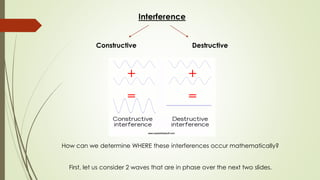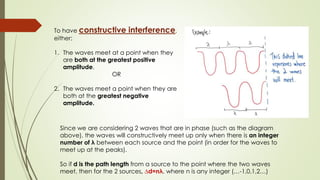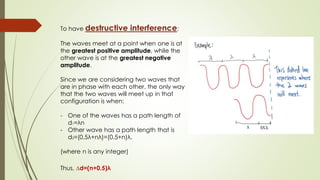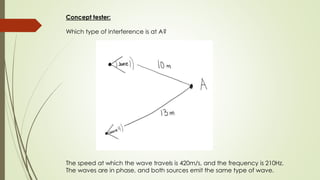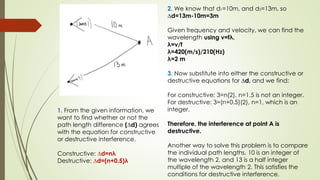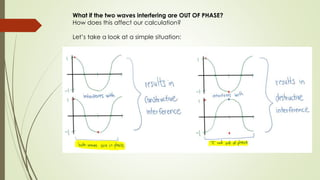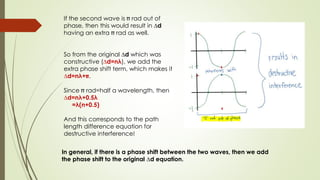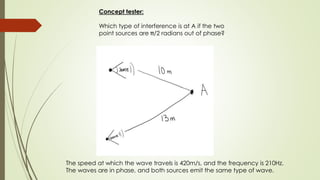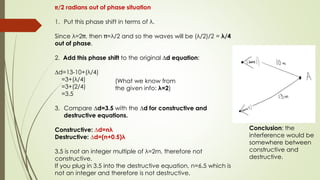1 of 10

### Learning object 7 (interference)

1. Exploring Interference By: Eugenie Kwong Learning Object 7 PHYS 101-202 Section LC2
2. Interference Constructive Destructive How can we determine WHERE these interferences occur mathematically? First, let us consider 2 waves that are in phase over the next two slides.
3. To have constructive interference, either: 1. The waves meet at a point when they are both at the greatest positive amplitude. OR 2. The waves meet a point when they are both at the greatest negative amplitude. Since we are considering 2 waves that are in phase (such as the diagram above), the waves will constructively meet up only when there is an integer number of λ between each source and the point (in order for the waves to meet up at the peaks). So if d is the path length from a source to the point where the two waves meet, then for the 2 sources, ∆d=nλ, where n is any integer (…-1,0,1,2…)
4. To have destructive interference: The waves meet at a point when one is at the greatest positive amplitude, while the other wave is at the greatest negative amplitude. Since we are considering two waves that are in phase with each other, the only way that the two waves will meet up in that configuration is when: - One of the waves has a path length of d1=λn - Other wave has a path length that is d2=(0.5λ+nλ)=(0.5+n)λ. (where n is any integer) Thus, ∆d=(n+0.5)λ
5. Concept tester: Which type of interference is at A? The speed at which the wave travels is 420m/s, and the frequency is 210Hz. The waves are in phase, and both sources emit the same type of wave.
6. 1. From the given information, we want to find whether or not the path length difference (∆d) agrees with the equation for constructive or destructive interference. Constructive: ∆d=nλ Destructive: ∆d=(n+0.5)λ 2. We know that d1=10m, and d2=13m, so ∆d=13m-10m=3m Given frequency and velocity, we can find the wavelength using v=fλ. λ=v/f λ=420(m/s)/210(Hz) λ=2 m 3. Now substitute into either the constructive or destructive equations for ∆d, and we find: For constructive: 3=n(2), n=1.5 is not an integer. For destructive: 3=(n+0.5)(2), n=1, which is an integer. Therefore, the interference at point A is destructive. Another way to solve this problem is to compare the individual path lengths. 10 is an integer of the wavelength 2, and 13 is a half integer multiple of the wavelength 2. This satisfies the conditions for destructive interference.
7. What if the two waves interfering are OUT OF PHASE? How does this affect our calculation? Let’s take a look at a simple situation:
8. If the second wave is rad out of phase, then this would result in ∆d having an extra rad as well. So from the original ∆d which was constructive (∆d=nλ), we add the extra phase shift term, which makes it ∆d=nλ+ . Since rad=half a wavelength, then ∆d=nλ+0.5λ =λ(n+0.5) And this corresponds to the path length difference equation for destructive interference! In general, if there is a phase shift between the two waves, then we add the phase shift to the original ∆d equation.
9. Concept tester: Which type of interference is at A if the two point sources are /2 radians out of phase? The speed at which the wave travels is 420m/s, and the frequency is 210Hz. The waves are in phase, and both sources emit the same type of wave.
10. /2 radians out of phase situation 1. Put this phase shift in terms of λ. Since λ=2 , then =λ/2 and so the waves will be (λ/2)/2 = λ/4 out of phase. 2. Add this phase shift to the original ∆d equation: ∆d=13-10+(λ/4) =3+(λ/4) =3+(2/4) =3.5 3. Compare ∆d=3.5 with the ∆d for constructive and destructive equations. Constructive: ∆d=nλ Destructive: ∆d=(n+0.5)λ 3.5 is not an integer multiple of λ=2m, therefore not constructive. If you plug in 3.5 into the destructive equation, n=6.5 which is not an integer and therefore is not destructive. (What we know from the given info: λ=2) Conclusion: the interference would be somewhere between constructive and destructive.